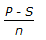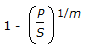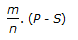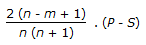# Chemical Engineering - Chemical Engineering Plant Economics

### Exercise :: Chemical Engineering Plant Economics - Section 1

36.

Pick out the wrong statement.

 A. Debt-equity ratio of a chemical company describes the lenders contribution for each rupee of owner's contribution i.e., debt-equity ratio = total debt/net worth. B. Return on investment (ROI) is the ratio of profit before interest & tax and capital employed (i.e. net worth + total debt). C. Working capital = current assets + current liability. D. Turn over = opening stock + production closing stock.

Explanation:

No answer description available for this question. Let us discuss.

37.

Personnel working in the market research group is reponsible for the job of

 A. equipment selection. B. product evaluation. C. equipment design. D. cost estimation.

Explanation:

No answer description available for this question. Let us discuss.

38.

"Break-even point" is the point of intersection of

 A. fixed cost and total cost. B. total cost and sales revenue. C. fixed cost and sales revenue. D. none of these.

Explanation:

No answer description available for this question. Let us discuss.

39.

'P' is the investment made on an equipment, 'S' is its salvage value and 'n is the life of the equipment in years. The depreciation for rath year by the sum-of years digit method will be

 A.B.C.D.Explanation:

No answer description available for this question. Let us discuss.

40.

Pick out the wrong statement.

 A. Gross revenue is that total amount of capital received as a result of the sale of goods or service. B. Net revenue is the total profit remaining after deducting all costs excluding taxes. C. The ratio of immediately available cash to the total current liabilities is known as the cash ratio. D. Consolidated income statement based on a given time period indicates surplus capital and shows the relationship among total income, costs & profit over the time interval.